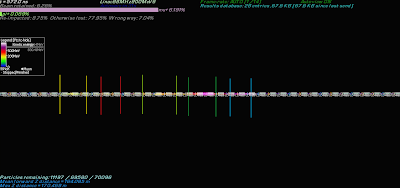## 2011-12-30

### Best Designs - Linac88MHz900MeV6

Just as Linac88MHz900MeV1 took PhaseRotLinac1 onward, so too will Linac88MHz900MeV6 for PhaserotLinac6.

The best design was 2.481781% by Team Anandtech's's amd.borg.

This was the fifth and final release in the January 2008 burst, but also had the most number of simulations performed. As the name implies, instead of the single block-per section of Linac88MHz900MeV1, it has 6.

This lattice is, like it's sister lattice, Linac88MHz900MeV1, a refinement. It is also notable because it's one of the first times you can clearly see the actions of the Phase Rotation in a simulation. I've marked the five distinct groups in this screengrab from the video at around the 3 minute mark.Phase Rotation groupings marked (click to enlarge)
The search space is in the 10375 range with 125 parameters. The lattice showed a single-stage growth pattern.

This is the 'best' design as recreated in v4.45. The original simulation length as recorded was 39 minutes 55 seconds.

The music is The Fire by Frank Harper released under a CC-BY-NC-SA license

Next time, Linac900NoGaps1DATA

Lattice
Linac88MHz900MeV6
Date of release
January 24nd, 2008
Total possible number of permutations
10475 (1 with 475 zeros after it)
Number of Simulations Run
666,864
Total MPTS
674,557,962.2
Target Energy Range
830.41MeV - 969.64MeV
Design

#gen=2;#runs=5;d1l=664;d2l=000;d3l=999;d4l=708;d5l=000;d6l=517;d7l=000;d8l=999;d9l=319;db1l=518;db2l=507;db3l=492;db4l=489;db5l=504;db6l=717;decaycells=000;ld1=000;ld2=000;ld3=000;ld4=000;ld5=417;ld6=433;linaccells=936;ls1f=000;ls1l=509;ls2f=000;ls2l=517;ls3f=000;ls3l=502;ls4f=000;ls4l=547;ls5f=000;ls5l=894;ls6f=992;ls6l=991;pd1=000;pd2=000;pd3=000;pd4=000;pd5=000;pd6=059;phaserotcells=000;prf1p=526;prf1v=990;prf2p=493;prf2v=999;prf3p=482;prf3v=999;prf4p=521;prf4v=998;prf5p=433;prf5v=999;prf6p=442;prf6v=999;ps1f=000;ps1l=625;ps2f=000;ps2l=610;ps3f=000;ps3l=622;ps4f=000;ps4l=587;ps5f=000;ps5l=560;ps6f=000;ps6l=598;rf1p=556;rf1v=999;rf2p=678;rf2v=999;rf3p=569;rf3v=999;rf4p=591;rf4v=996;rf5p=795;rf5v=999;rf6p=513;rf6v=999;s1f=998;s1l=569;s2f=000;s2l=790;s2r=680;s3f=920;s3l=304;s3r=787;s4f=105;s4l=677;s4r=412;s5f=000;s5l=126;s5r=381;s6f=000;s6l=823;s6r=703;s7f=023;s7l=084;s7r=799;s8f=000;s8l=936;s8r=999;s9f=046;s9l=826;s9r=733;sb1f=980;sb1l=896;sb1r=999;sb2f=987;sb2l=692;sb2r=935;sb3f=999;sb3l=689;sb3r=975;sb4f=946;sb4l=679;sb4r=999;sb5f=999;sb5l=743;sb5r=900;sb6f=999;sb6l=803;sb6r=658;tantalumrodr=000;tantalumrodz=130;
2.481781 (6466.5 Mpts) [v4.44d] <Linac88MHz900MeV6> by [TA]amd.borg